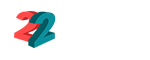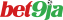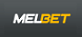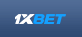# How Double Chance bets are calculated?

## what is the formula to calculate double chance bets?

 Top Online Bookmakers See All Betting SItes
1€122 Free bets
2₦100,000 Bonus
3Ksh 34,500 Free
4200% Welcome Bonus
5150\$ Welcome BonusIntro for How Double Chance bets are calculated?: what is the formula to calculate double chance bets?
• What are Double Chance Bets?
• Use the odds to calculate the stakes for Double Chance bets
• Betting Odds Double chance against a bookmaker's odds for winning or drawing

### What are Double Chance Bets?

Basically, Double chance bets offer the possibility to bet on two results of a match, eliminating the third option: that is, it is possible to bet on the home team and on the tie or on the away team and on the tie. Instead, the drawback option gives you a lower odds on the winning team and simply refunds the bet if the game ends in a draw.

Bookmakers often offer this kind of market, but is it possible to get a better value by calculating their odds Double chance bets using 1X2 odds?

On Pinnacle a bet with a handicap of +0.50 would work perfectly as a double chance bet. However, we do not offer 0 and 0.5 handicaps for all games, so it may be worth knowing how to calculate your Double chance bets odds.

Double chance bets are used in different sports especially football games. The following are the three formulas to calculate double chance bets. The first formula is when you want to calculate '1X' games. (Odds of Home Team * Odds of Draw) / (Odds of Home Team + Odds of Draw); so if the Odds of Home Team is 1.8 and Odds for draw are 3.3 the result will be (1.8 * 3.3) / ( 1.8 + 3.3)=> 1.17 (always rounded up to 2 decimal places).
The first formula is when you want to calculate '12' games. (Odds of Home Team * Odds of Away Team) / (Odds of Home Team + Odds of Away Team); so if the Odds of Home Team is 1.8 and Odds of Away Team are 6.2 the result will be (1.8 * 6.2) / ( 1.8 + 6.2)=> 1.4 (always rounded up to 2 decimal places).
The first formula is when you want to calculate '12' games. (Odds of Draw * Odds of Away Team) / (Odds of Draw + Odds of Away Team); so if the Odds of Draw is 3.3 and Odds of Away Team are 6.2 the result will be (3.3 * 6.2) / (3.3 + 6.2)=> 2.2 (always rounded up to 2 decimal places).Other Pages you may like:

Casino & Sports Links on Feedinco